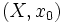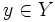# Difference between revisions of "Applying path-connectedness"

This is a survey article about applying the concept/definition/theorem: path-connectedness

The hypothesis of being path-connected is in general stronger than the hypothesis of being connected, although for locally path-connected spaces, such as manifolds, the two hypotheses are equivalent. In certain situations, connectedness is a more useful hypothesis, while in other situations, it is more useful to use path-connectedness. Refer the article on applying connectedness.

## Constructing paths and homotopies

The most useful and direct application of path-connectedness is to construct paths, which can be used to define homotopies and change basepoints.

### Basepoint-independence of homotopy groups

The homotopy groups of a topological space are usually defined with respect to a basepoint; in order to prove that the homotopy groups at two basepoints are isomorphic, we use a path between them to give an isomorphism. In particular, the fundamental groups at any two basepoints in a path-connected space are the same. Moreover, if$Y$ is a path-connected space, and$X$ is a space with nondegenerate basepoint$x_0$, then the homotopy classes of based maps from$(X,x_0)$ to$(Y,y)$ can be identified for different choices of basepoint$y \in Y$.

Further information: Actions of the fundamental group

### Multiplication maps in a group

In a path-connected topological group, the multiplication map by any group element is homotopy-equivalent to the identity map. The homotopy in this case is given by the path joining the group element to the identity element. In particular, any map given by an algebraic formula is homotopy-equivalent to a power map.

This fact is useful, for instance, in showing that any compact connected nontrivial Lie group has zero Euler characteristic. The idea is that the fixed-point free map of left multiplication by a group element, is homotopy-equivalent to the identity map.

### Constructing more complicated homotopies

A homotopy can be viewed as a kind of generalized path, and we can often use a path, or a loop, as the starting point of a homotopy. The typical additional concepts we need are those of a cofibration, and we often need to use tricks such as the two sides lemma or three sides lemma.